# Drawing a custom rating view in SwiftUI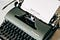Photo by Markus Winkler on Unsplash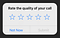WhatsApp surveying call quality using ratings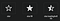Star symbol in SFSymbols

# Getting Started

## 1. Create new SwiftUI project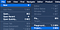Create a new Xcode project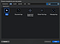Select the App template from the iOS tab

## 2. Create star shape

`struct Star: Shape {    ...}`
`let sides: Int = 5`
`func path(in rect: CGRect) -> Path`
`func degree2Radian(_ degree: CGFloat) -> CGFloat {    return CGFloat.pi * degree/180}func polygonPointArray(sides: Int,                       x: CGFloat,                       y: CGFloat,                       radius: CGFloat,                       adjustment: CGFloat = 0) -> [CGPoint] {    let angle = degree2Radian(360/CGFloat(sides))    return Array(0...sides).map({ side -> CGPoint in        let adjustedAngle: CGFloat = angle * CGFloat(side) + degree2Radian(adjustment)        let xpo = x - radius * cos(adjustedAngle)        let ypo = y - radius * sin(adjustedAngle)        return CGPoint(x: xpo, y: ypo)    })}`
`var path = Path()let startAngle = CGFloat(-1*(360/sides/4))let adjustment = startAngle + CGFloat(360/sides/2)let center = CGPoint(x: rect.width/2, y: rect.height/2)let innerPolygon = polygonPointArray(sides: self.sides,                                     x: center.x,                                     y: center.y,                                     radius: rect.width/5,                                     adjustment: startAngle)let outerPolygon = polygonPointArray(sides: self.sides,                                     x: center.x,                                     y: center.y,                                     radius: rect.width/2,                                     adjustment: adjustment)let points = zip(innerPolygon, outerPolygon)path.move(to: innerPolygon)points.forEach({ (innerPoint, outerPoint) in    path.addLine(to: innerPoint)    path.addLine(to: outerPoint)})path.closeSubpath()return path`
`Star()    .fill(Color(UIColor.systemGray4))    .background(Color.green)    .frame(width: 50, height: 50, alignment: .center)`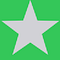Star preview

## 3. Create a star view

`let fillAmount: CGFloat`
`ZStack {    Rectangle()        .fill(Color.gray)    GeometryReader { geometry in        Rectangle()            .fill(Color.red)            .frame(width: geometry.size.width * fillAmount, height: geometry.size.height, alignment: .leading)    }}`
`struct StarView_Previews: PreviewProvider {    static var previews: some View {        StarView(fillAmount: 0.2)            .frame(width: 50, height: 50, alignment: .center)    }}`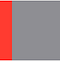StarVIew preview
`ZStack {    ...}.mask(Star())`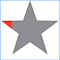StarView preview

## 4. Display average ratings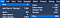Create a new file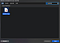Search and select SwiftUI View
`HStack {    StarView(fillAmount: 1.0)    StarView(fillAmount: 1.0)    StarView(fillAmount: 1.0)    StarView(fillAmount: 1.0)    StarView(fillAmount: 0.2)}`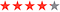Now displaying 5 stars
`@Binding var rating: CGFloat`
`private func getFillAmount(forIndex index: Int) -> CGFloat {    let calc = self.rating - CGFloat(index)    if calc >= 0.0 {        return 1.0    } else if calc <= 0.0 && calc > -1.0 {        return self.rating - CGFloat(index - 1)    } else {        return 0.0    }}`
`HStack {    StarView(fillAmount: self.getFillAmount(forIndex: 1))    StarView(fillAmount: self.getFillAmount(forIndex: 2))    StarView(fillAmount: self.getFillAmount(forIndex: 3))    StarView(fillAmount: self.getFillAmount(forIndex: 4))    StarView(fillAmount: self.getFillAmount(forIndex: 5))}`
`struct StatefulPreviewWrapper<Value, Content: View>: View {    @State var value: Value    var content: (Binding<Value>) -> Content    var body: some View {        content(\$value)    }    init(_ value: Value, content: @escaping (Binding<Value>) -> Content) {        self._value = State(wrappedValue: value)        self.content = content    }}`
`StatefulPreviewWrapper(4.2) { RatingsView(rating: \$0) }`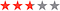Average rating of 2.7

## 5. Allow the user to rate

`var onDidRate: ((Int) -> ())?`
`private func didRate(_ rate: Int) {    self.onDidRate?(rate)}`
`HStack {    StarView(fillAmount: self.getFillAmount(forIndex: 1)).onTapGesture(count: 1, perform: {        self.didRate(1)    })    StarView(fillAmount: self.getFillAmount(forIndex: 2)).onTapGesture(count: 1, perform: {        self.didRate(2)    })    StarView(fillAmount: self.getFillAmount(forIndex: 3)).onTapGesture(count: 1, perform: {        self.didRate(3)    })    StarView(fillAmount: self.getFillAmount(forIndex: 4)).onTapGesture(count: 1, perform: {        self.didRate(4)    })    StarView(fillAmount: self.getFillAmount(forIndex: 5)).onTapGesture(count: 1, perform: {        self.didRate(5)    })}`
`@State var userRating: CGFloat = 0.0@State var isUserRating = false@State var averageRating: CGFloat = 4.2@State var totalRatings = 34`
`private func userDidRate(_ rating: Int) {    let newRating = CGFloat(rating)    self.userRating = newRating    let currentNumberOfRatings = self.totalRatings    self.totalRatings += 1    self.averageRating = ((self.averageRating * CGFloat(currentNumberOfRatings)) + newRating) / CGFloat(self.totalRatings)    DispatchQueue.main.asyncAfter(deadline: .now() + 1.0, execute: { self.isUserRating = false })}`
`private func getRatingsSummary() -> String {    let averageRatingToOneDecimalPlace = String(format: "%.1f", self.averageRating)    return "\(averageRatingToOneDecimalPlace) average • \(self.totalRatings) ratings"}`
`if self.isUserRating {    VStack {        RatingsView(onDidRate: self.userDidRate, rating: self.\$userRating)            .frame(width: .infinity, height: 100, alignment: .center)        Text("Rate it!")    }} else {    VStack {        RatingsView(rating: self.\$averageRating)            .frame(width: .infinity, height: 100, alignment: .center)        Text(self.getRatingsSummary())        if userRating == 0.0 {            Button("Rate") {                self.isUserRating = true            }        }    }}`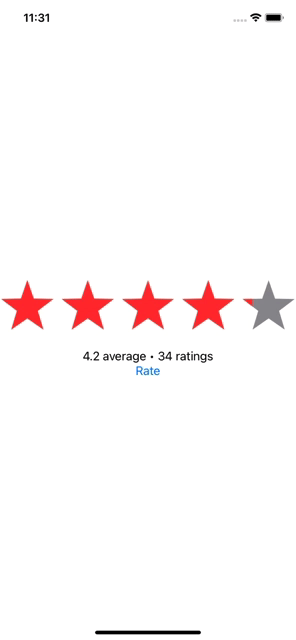RatingsView in action

# Final Notes

Senior iOS Engineer @ Onfido. Writing weekly blogs on iOS and programming. Follow me to stay tuned!